﻿ 波流耦合模式及其在理想潮汐通道中的应用
«上一篇文章快速检索 高级检索

 哈尔滨工程大学学报2019, Vol. 40Issue (8): 1420-1426  DOI: 10.11990/jheu.2018051270

### 引用本文WU Zhiyuan, JIANG Changbo, DENG Bin, et al. SWAN-ROMS coupling model and its application in idealized tidal inlets[J]. Journal of Harbin Engineering University, 2019, 40(8), 1420-1426. DOI: 10.11990/jheu.201805127.### 文章历史

1. 长沙理工大学 水利工程学院, 湖南 长沙 410004;
2. 水沙科学与水灾害防治湖南省重点实验室, 湖南 长沙 410004;
3. 美国麻省大学 海洋科学与技术学院, 美国马萨诸塞州 新贝德福德 02744;
4. 国家海洋局 南海调查技术中心, 广东 广州 510300

SWAN-ROMS coupling model and its application in idealized tidal inlets
WU Zhiyuan 1,2,3, JIANG Changbo 1,2, DENG Bin 1,2, CAO Yonggang 4
1. School of Hydraulic Engineering, Changsha University of Science & Technology, Changsha 410004, China;
2. Hu'nan Provincial Key Laboratory of Water, Sediment Sciences & Flood Hazard Prevention, Changsha 410004, China;
3. School for Marine Science and Technology, University of Massachusetts Dartmouth, New Bedford, MA 02744, USA;
4. Key Laboratory of Technology for Safeguarding of Maritime Rights and Interests and Application, State Oceanic Administration, Guangzhou 510300, China
Abstract: Waves and currents are the two most important dynamic factors in coastal areas. The interaction between waves and currents is highly complex. In this study, the model coupling toolkit is used to couple the regional ocean models (ROMS) with the third-generation wave and current Simulating Waves Nearshore (SWAN) model to establish the real time, two-way coupling SWAN-ROMS model. Typical examples of ideal tidal channels are subjected to wave-current coupling calculation and analysis. Numerical experiments are performed by using the SWAN model alone and by using the coupled SWAN-ROMS model. Then, these two schemes are compared to analyze the influence on waves and tides in wave-current interaction. Results show that the wave-current coupling model can simulate the wave-current interaction in tidal inlets well. Moreover, results show that the existence of tidal current has drastic effects on waves and that periodic current movements cause the steady wave field to change periodically. Wave height will decrease when tidal currents and waves propagate in the same direction and will decrease when tidal currents and waves propagate in opposite directions.
Keywords: wave-current interaction    tidal inlet    ocean modeling    wave modeling    radiation stress

1 数学模型简介 1.1 区域海洋模式

 $\begin{array}{*{20}{c}} {\frac{{\partial \left( {{H_z}u} \right)}}{{\partial t}} + \frac{{\partial \left( {u{H_z}u} \right)}}{{\partial x}} + \frac{{\partial \left( {v{H_z}u} \right)}}{{\partial y}} + \frac{{\partial \left( {w{H_z}u} \right)}}{{\partial s}} - }\\ {f{H_z}v = - \frac{{{H_z}}}{{{\rho _0}}}\frac{{\partial p}}{{\partial x}} - {H_z}g\frac{{\partial \eta }}{{\partial x}} - \frac{\partial }{{\partial s}}\left( {\overline {u'w'} - \frac{v}{{{H_z}}}\frac{{\partial u}}{{\partial s}}} \right) - }\\ {\frac{{\partial \left( {{H_z}{S_{xx}}} \right)}}{{\partial x}} - \frac{{\partial \left( {{H_z}{S_{xy}}} \right)}}{{\partial y}} + \frac{{\partial {S_{px}}}}{{\partial s}}} \end{array}$ (1)
 $\begin{array}{*{20}{c}} {\frac{{\partial \left( {{H_z}v} \right)}}{{\partial t}} + \frac{{\partial \left( {u{H_z}v} \right)}}{{\partial x}} + \frac{{\partial \left( {v{H_z}v} \right)}}{{\partial y}} + \frac{{\partial \left( {w{H_z}v} \right)}}{{\partial s}} - }\\ {f{H_z}u = - \frac{{{H_z}}}{{{\rho _0}}}\frac{{\partial p}}{{\partial y}} - {H_z}g\frac{{\partial \eta }}{{\partial y}} - \frac{\partial }{{\partial s}}\left( {\overline {v'w'} - \frac{v}{{{H_z}}}\frac{{\partial v}}{{\partial s}}} \right) - }\\ {\frac{{\partial \left( {{H_z}{S_{yx}}} \right)}}{{\partial x}} - \frac{{\partial \left( {{H_z}{S_{yy}}} \right)}}{{\partial y}} + \frac{{\partial {S_{py}}}}{{\partial s}}} \end{array}$ (2)
 $- \frac{1}{{{\rho _0}}}\frac{{\partial p}}{{\partial s}} - \frac{g}{{{\rho _0}}}{H_z}\rho = 0$ (3)

 $\frac{{\partial \eta }}{{\partial t}} + \frac{{\partial \left( {{H_z}u} \right)}}{{\partial x}} + \frac{{\partial \left( {{H_z}v} \right)}}{{\partial y}} + \frac{{\partial \left( {{H_z}w} \right)}}{{\partial s}} = 0$ (4)

 $\begin{array}{*{20}{c}} {\frac{{\partial \left( {{H_z}C} \right)}}{{\partial t}} + \frac{{\partial \left( {u{H_z}C} \right)}}{{\partial x}} + \frac{{\partial \left( {v{H_z}C} \right)}}{{\partial y}} + \frac{{\partial \left( {w{H_z}C} \right)}}{{\partial s}} = }\\ { - \frac{\partial }{{\partial s}}\left( {\overline {c'w'} - \frac{{{v_\theta }}}{{{H_z}}}\frac{{\partial C}}{{\partial s}}} \right) + {C_{{\rm{source}}}}} \end{array}$ (5)

 $\rho = f\left( C \right)$ (6)

1.2 第3代海浪模式SWAN

1.3 三维辐射应力计算方法

 $\left\{ \begin{array}{l} {S_{xx}} = kE\left( {\frac{{{k_x}{k_x}}}{{{k^2}}}{F_{CS}}{F_{CC}} + {F_{CC}}{F_{CS}} - {F_{SS}}{F_{CS}}} \right) + \frac{{{k_x}{k_x}}}{k}\frac{{{c^2}}}{L}{A_R}{R_z}\\ {S_{xy}} = {S_{yx}} = kE\left( {\frac{{{k_x}{k_y}}}{{{k^2}}}{F_{CS}}{F_{CC}}} \right) + \frac{{{k_x}{k_y}}}{k}{A_R}{R_z}\\ {S_{yy}} = kE\left( {\frac{{{k_y}{k_y}}}{{{k^2}}}{F_{CS}}{F_{CC}} + {F_{CS}}{F_{CC}} - {F_{SS}}{F_{CS}}} \right) + \frac{{{k_y}{k_y}}}{k}\frac{{{c^2}}}{L}{A_R}{R_z} \end{array} \right.$ (7)

 $R_{z}=1-\tanh (2 s / \gamma)^{4}$ (8)

 $A_{\mathrm{R}}=\alpha H_{s} L Q_{b} / \sqrt{2}$ (9)

 $\left\{ \begin{array}{l} {S_{px}} = \left( {{F_{CC}} - {F_{SS}}} \right)\left[ {\frac{{{F_{SS}}}}{2}\frac{{\partial E}}{{\partial x}} + {F_{CS}}(1 + s)E\frac{{\partial (kD)}}{{\partial x}} - } \right.\\ \;\;\;\;\;\;\;\;\;\left. {E{F_{SS}}\coth (kD)\frac{{\partial (kD)}}{{\partial x}}} \right]\\ {S_{py}} = \left( {{F_{CC}} - {F_{SS}}} \right)\left[ {\frac{{{F_{SS}}}}{2}\frac{{\partial E}}{{\partial y}} + {F_{CS}}(1 + s)E\frac{{\partial (kD)}}{{\partial y}} - } \right.\\ \;\;\;\;\;\;\;\;\;\left. {E{F_{SS}}\coth (kD)\frac{{\partial (kD)}}{{\partial y}}} \right] \end{array} \right.$ (10)

 $\left\{ \begin{array}{l} {F_{SS}} = \frac{{\sinh \left[ {kD\left( {1 + s} \right)} \right]}}{{\sinh \left( {kD} \right)}},{F_{CS}} = \frac{{\cosh \left[ {kD\left( {1 + s} \right)} \right]}}{{\sinh \left( {kD} \right)}}\\ {F_{SC}} = \frac{{\sinh \left[ {kD\left( {1 + s} \right)} \right]}}{{\cosh \left( {kD} \right)}},{F_{CC}} = \frac{{\cosh \left[ {kD\left( {1 + s} \right)} \right]}}{{\cosh \left( {kD} \right)}} \end{array} \right.$ (11)

ROMS在解方程时使用了内外模式分别求解的方法，这种方法需要求解垂向平均的动量方程。包含辐射应力项的垂向平均动量方程为：

 $\begin{array}{*{20}{c}} {\frac{{\partial (D\bar u)}}{{\partial t}} + \frac{{\partial (\bar uD\bar u)}}{{\partial x}} + \frac{{\partial (\bar vD\bar v)}}{{\partial y}} - fD\bar v = - D\frac{{\partial p}}{{\partial x}} + }\\ {{\tau _{sx}} - {\tau _{bx}} - \frac{{\partial {{\bar S}_{xx}}}}{{\partial x}} - \frac{{\partial {{\bar S}_{xy}}}}{{\partial y}}} \end{array}$ (12)
 $\begin{array}{*{20}{c}} {\frac{{\partial (D\bar v)}}{{\partial t}} + \frac{{\partial (\bar vD\bar v)}}{{\partial x}} + \frac{{\partial (\bar vD\bar v)}}{{\partial y}} - fD\bar u = - D\frac{{\partial p}}{{\partial y}} + }\\ {{\tau _{sy}} - {\tau _{by}} - \frac{{\partial {{\bar S}_{xy}}}}{{\partial x}} - \frac{{\partial {{\bar S}_{yy}}}}{{\partial y}}} \end{array}$ (13)

 $\frac{{\partial \eta }}{{\partial t}} + \frac{{\partial \left( {D\bar u} \right)}}{{\partial x}} + \frac{{\partial \left( {D\bar v} \right)}}{{\partial y}} = 0$ (14)

 $\left\{ \begin{array}{l} {{\bar S}_{xx}} = E\frac{{{c_g}}}{c}\frac{{{k_x}{k_x}}}{{{k^2}}} + E\left( {\frac{{{c_g}}}{c} - \frac{1}{2}} \right) + \frac{{{k_x}{k_x}}}{k}\frac{{{c^2}{A_R}}}{L}\\ {{\bar S}_{xy}} = {{\bar S}_{yx}} = E\frac{{{c_g}}}{c}\frac{{{k_x}{k_y}}}{{{k^2}}} + \frac{{{k_x}{k_y}}}{k}\frac{{{c^2}{A_R}}}{L}\\ {{\bar S}_{yy}} = E\frac{{{c_g}}}{c}\frac{{{k_y}{k_y}}}{{{k^2}}} + E\left( {\frac{{{c_g}}}{c} - \frac{1}{2}} \right) + \frac{{{k_y}{k_y}}}{k}\frac{{{c^2}{A_R}}}{L} \end{array} \right.$ (15)

 $c_{g}=\frac{\partial \sigma}{\partial k}=\frac{c}{2}\left[1+\frac{2 k D}{\sinh (2 k D)}\right]$ (16)

 ${\bar u_s} = \frac{{{k_x}E}}{{ckD}} + \frac{{{k_x}}}{k}\frac{{g{A_R}}}{{cL}},{\bar v_s} = \frac{{{k_y}E}}{{ckD}} + \frac{{{k_y}}}{k}\frac{{g{A_R}}}{{cL}}$ (17)

1.4 波流实时双向耦合方法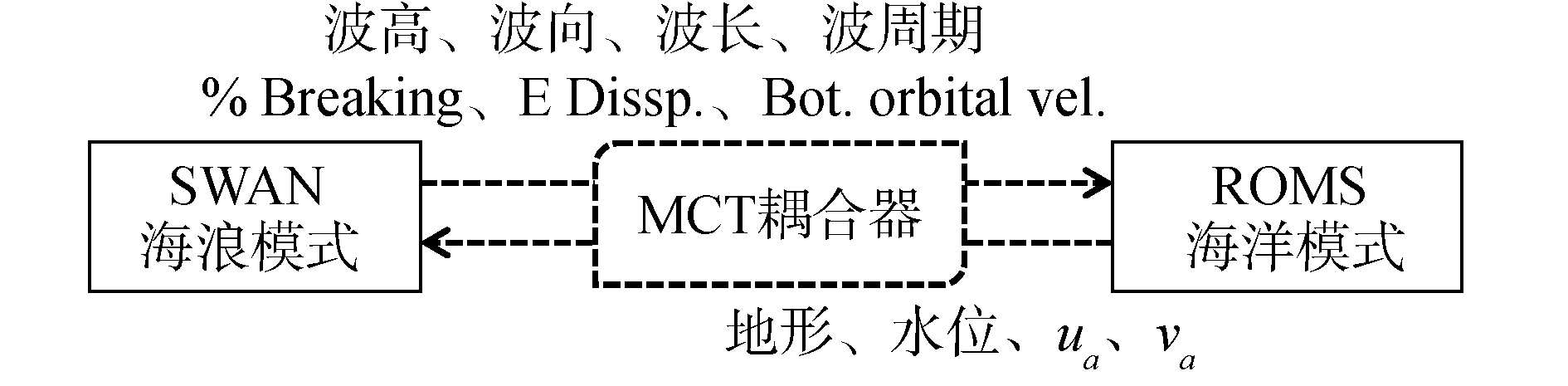Download: 图 1 海浪—海洋耦合机制及数据传递示意图 Fig. 1 Diagrammatic sketch of coupling mechanism and data switching in wave and ocean model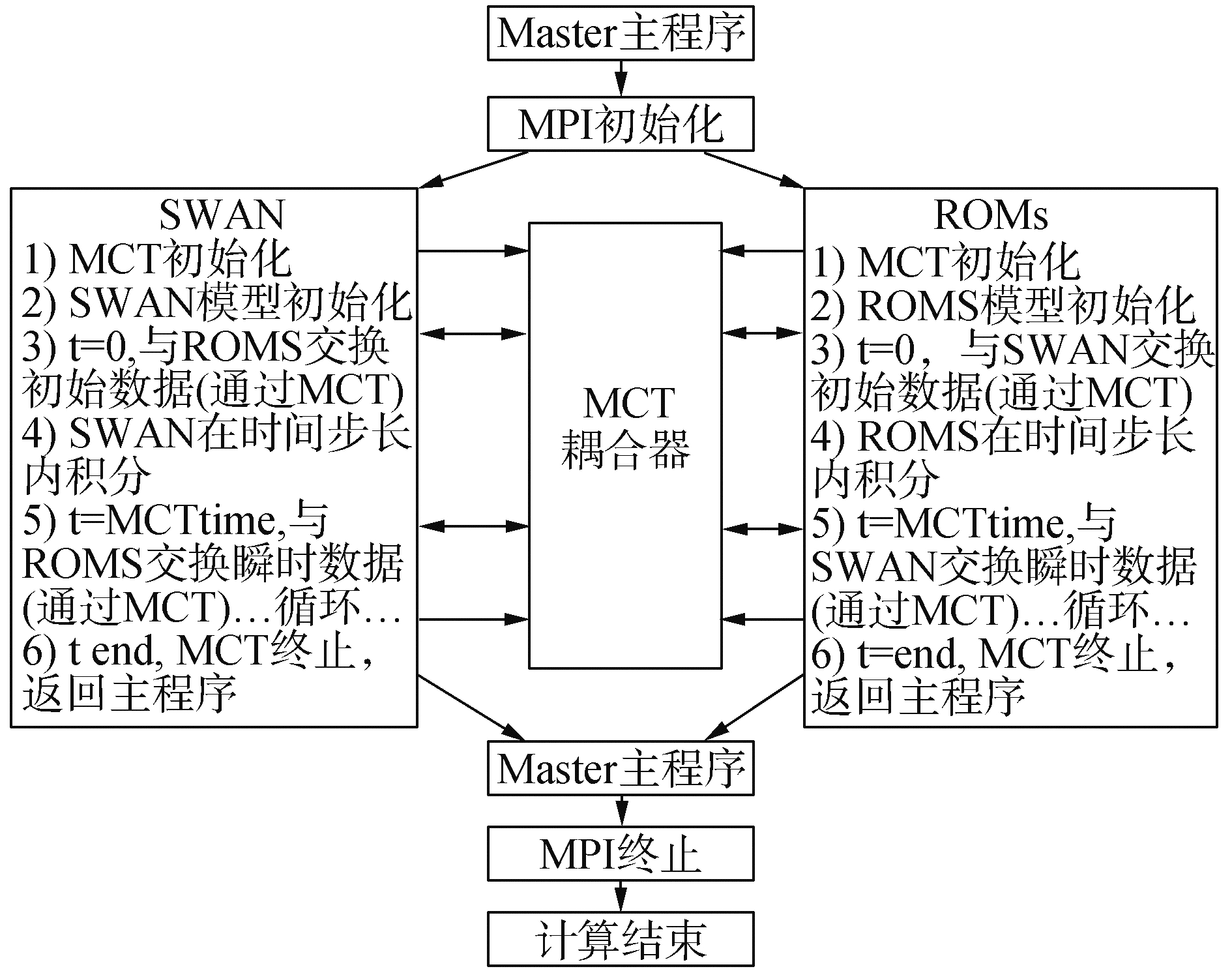Download: 图 2 基于MCT耦合的SWAN-ROMS模式计算流程示意图 Fig. 2 Diagrammatic sketch of calculation process in coupled SWAN-ROMS model
2 波流耦合模型的应用 2.1 模型设置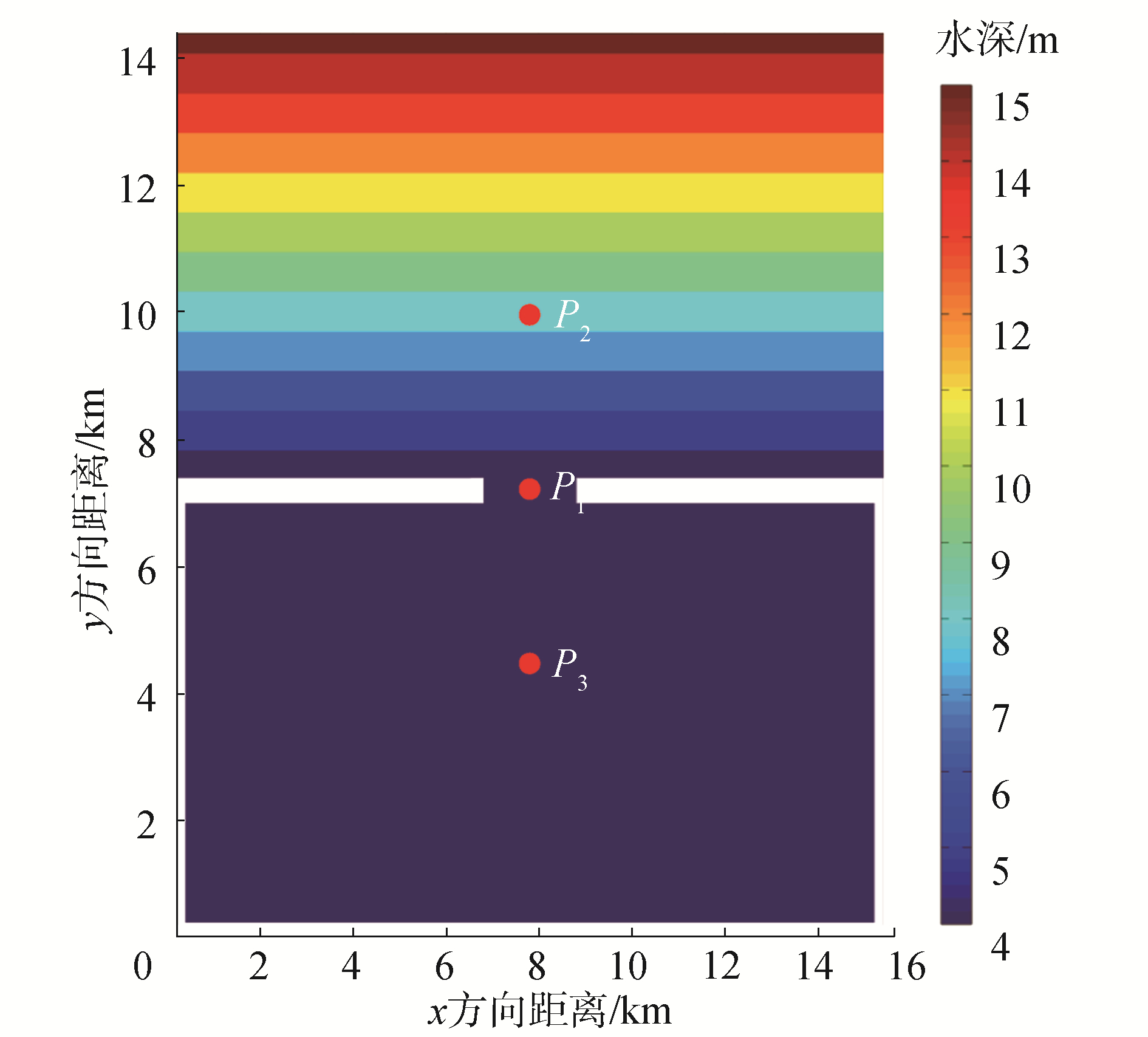Download: 图 3 理想潮汐通道算例水深分布 Fig. 3 Topography of the idealized tidal inlet

2.2 波高模拟结果分析 2.2.1 无潮流影响下的波高分布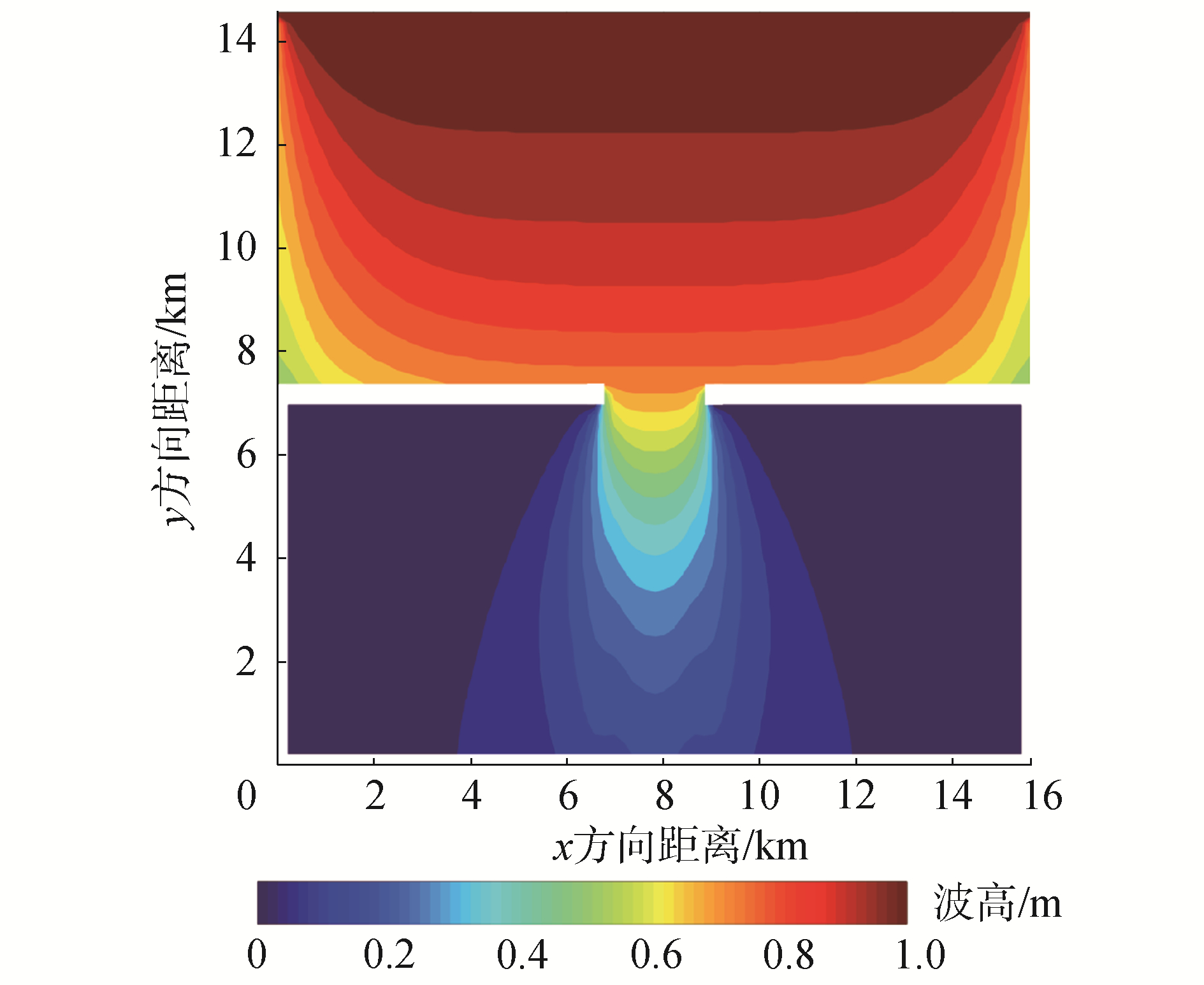Download: 图 4 无潮流影响下波高分布(t=6 h) Fig. 4 Distribution of wave height in the Exp1 (t=6 h)

2.2.2 潮流对波高分布的影响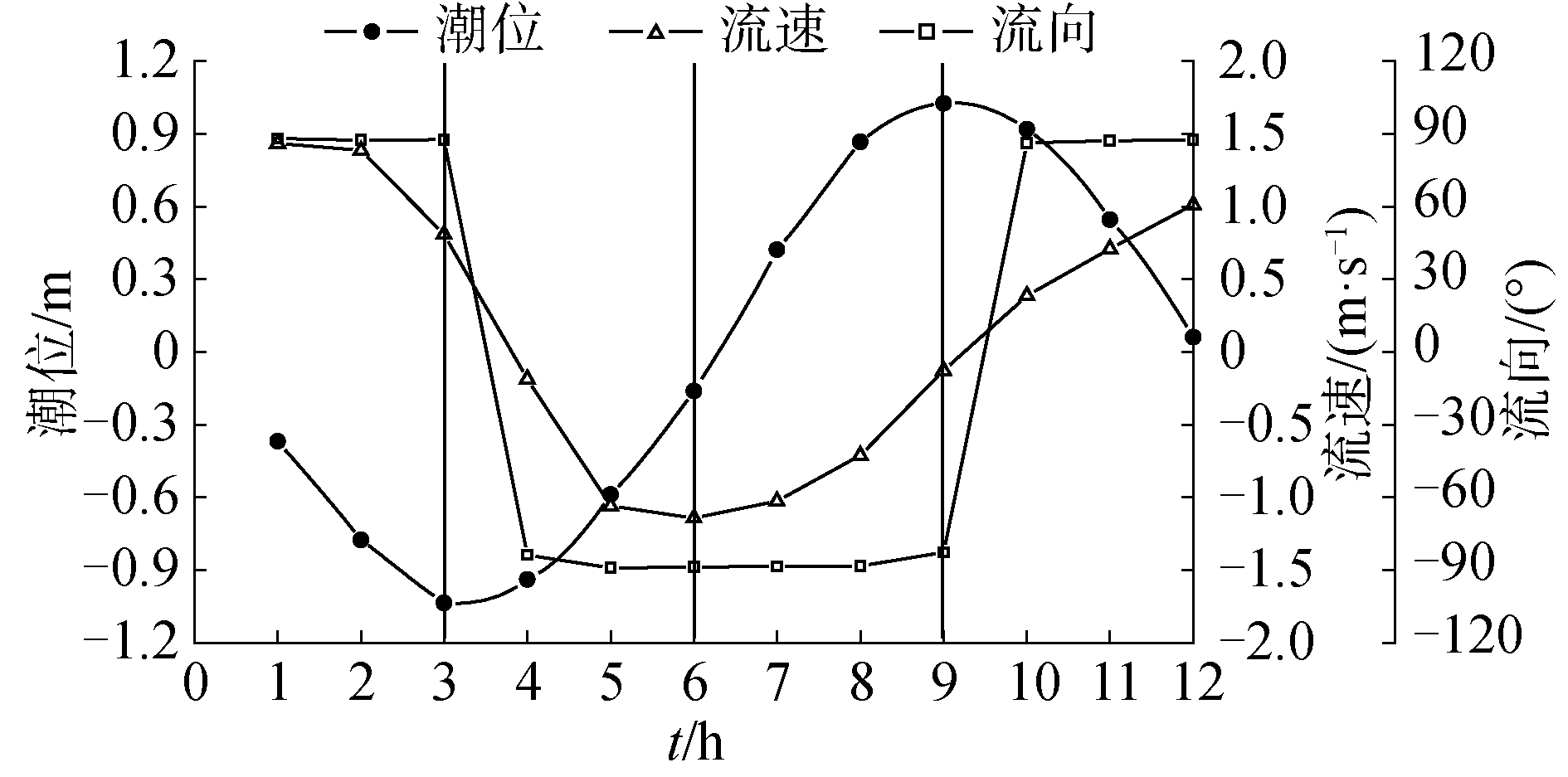Download: 图 5 潮汐通道中间P1点潮位、流速及流向过程 Fig. 5 Tidal level, velocity and direction of P1 in the inletDownload: 图 6 波流耦合模式下不同时刻波高及流速矢量分布 Fig. 6 Distribution of wave height and flow field in the Exp2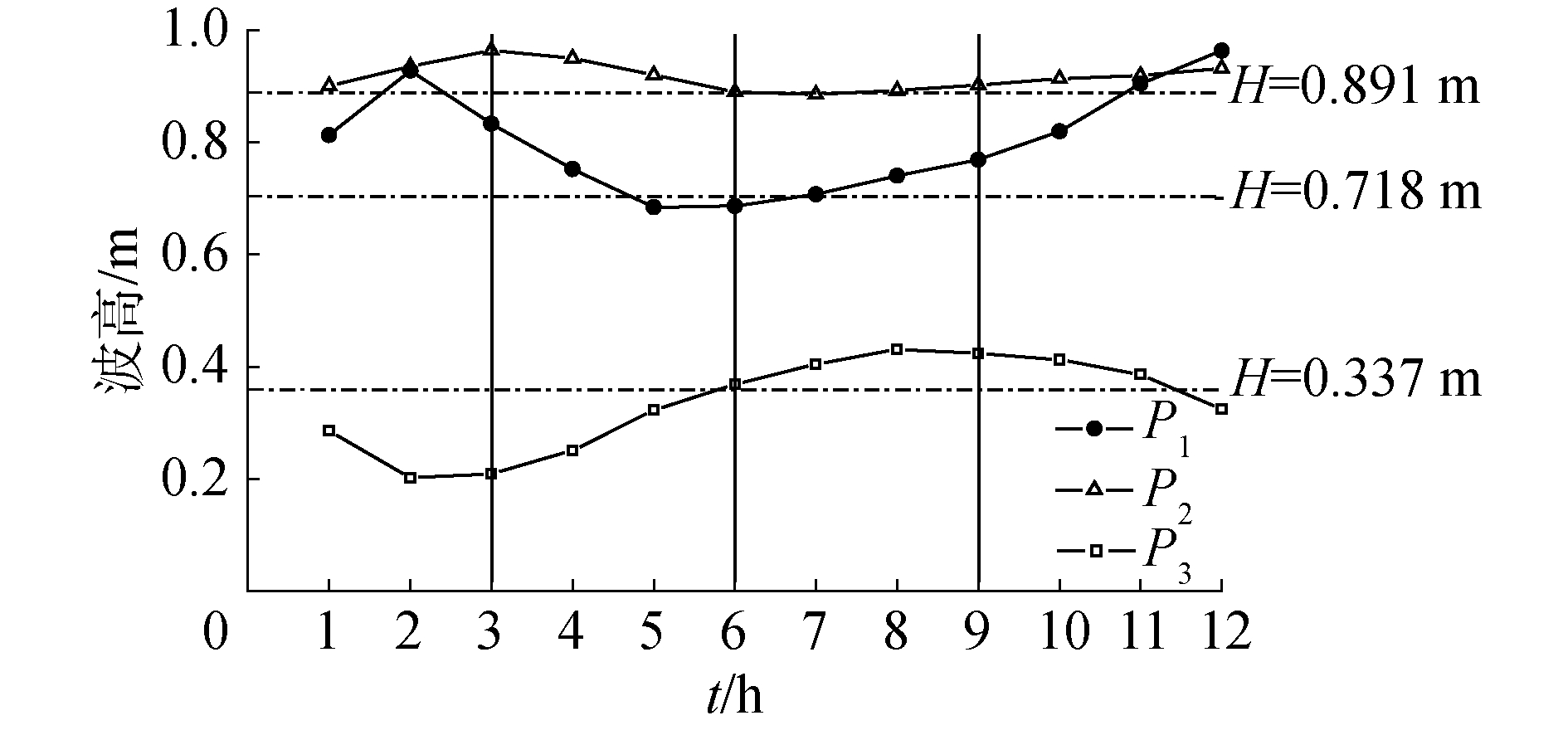Download: 图 7 各特征点波高随时间变化关系 Fig. 7 The impact-time history curves of wave height in three characteristic positions

2.3 潮流模拟结果分析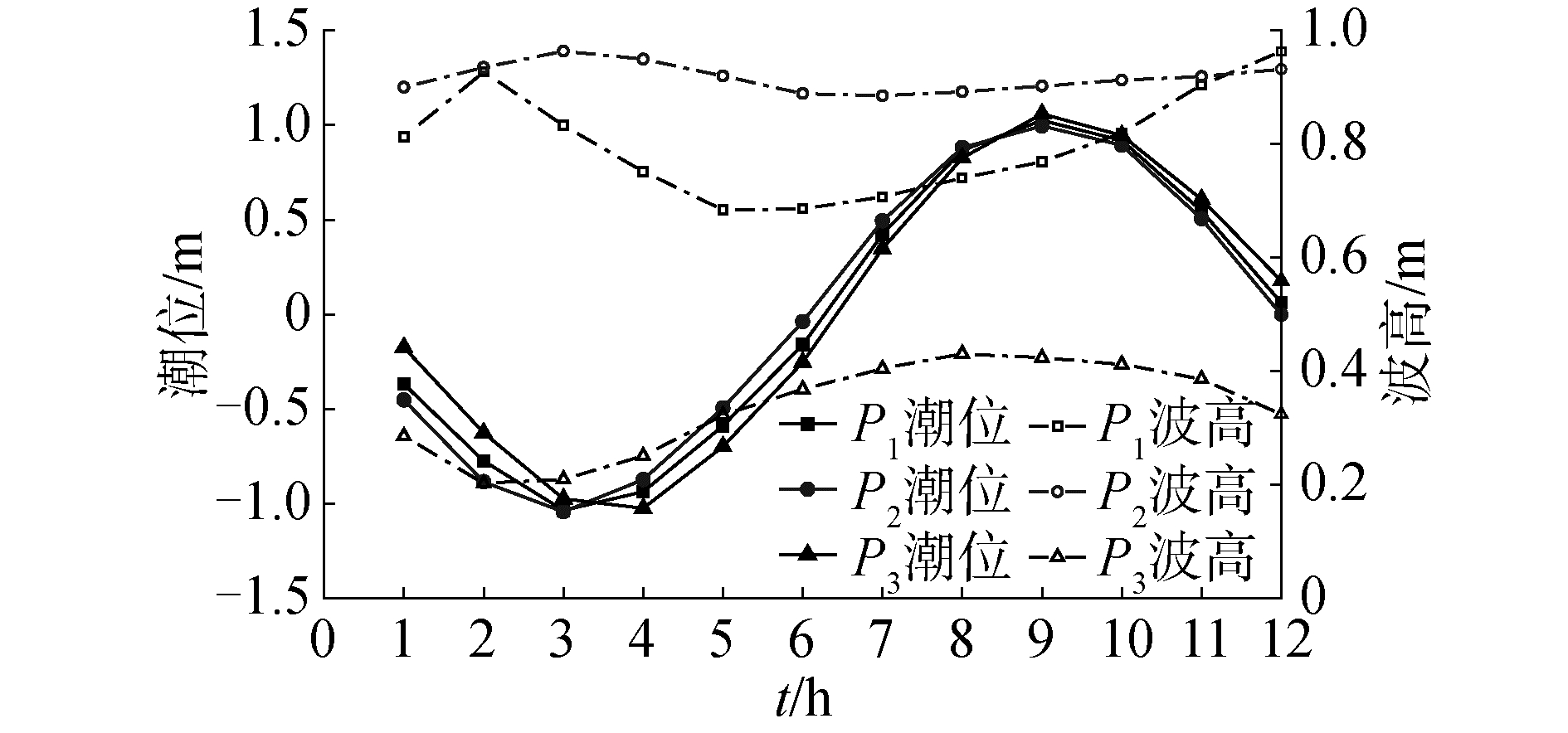Download: 图 8 各特征点水位、波高随时间变化关系 Fig. 8 The impact-time history curves of tidal level and wave height in three characteristic positionsDownload: 图 9 波流耦合模式下不同时刻表层流速分布 Fig. 9 Distribution of flow field in surface in the Exp2Download: 图 10 波流耦合模式下不同时刻底层流速分布 Fig. 10 Distribution of flow field in bottom in the Exp2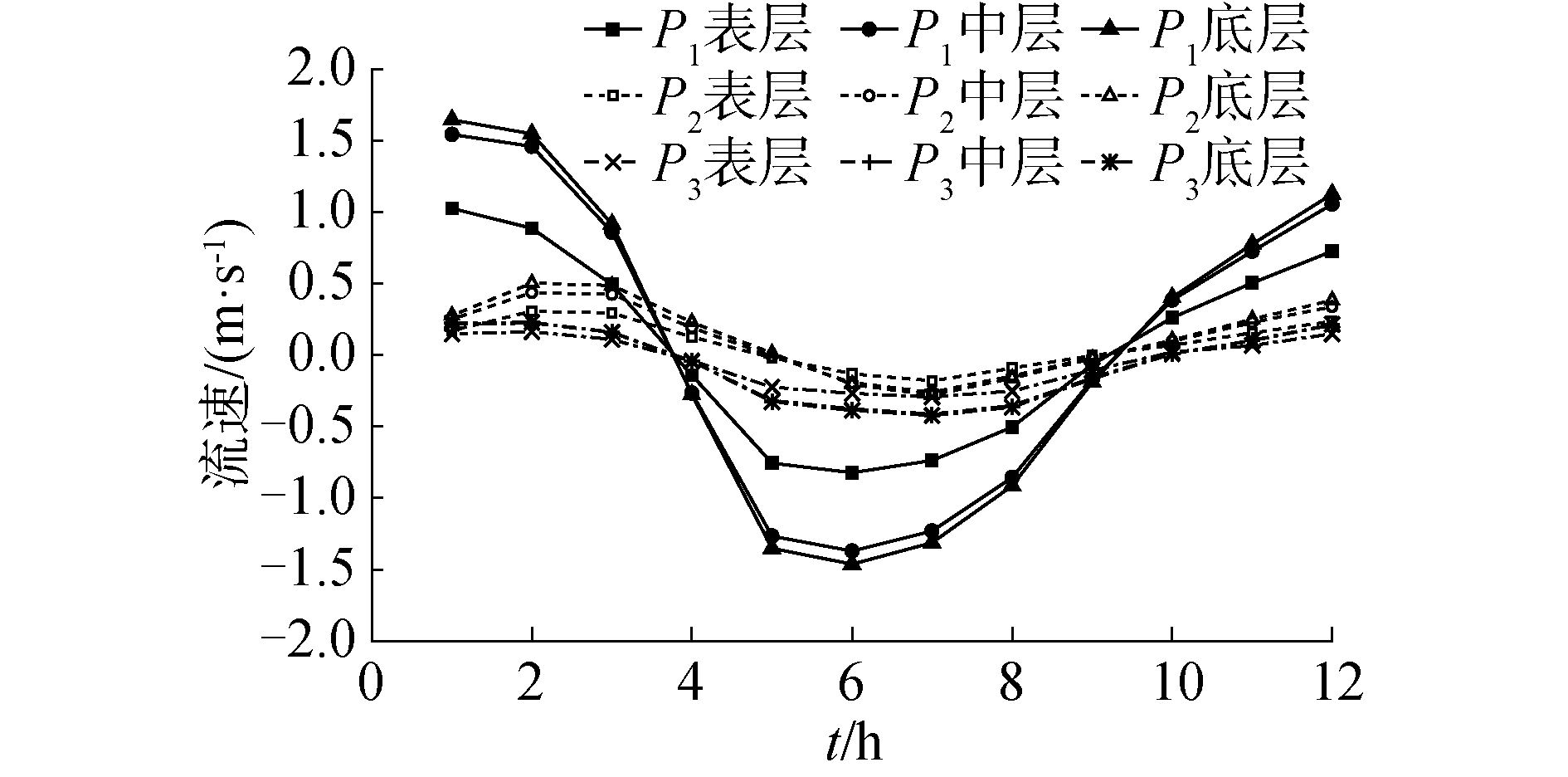Download: 图 11 各特征位置分层流速随时间的变化关系 Fig. 11 The impact-time history curves of current velocity on the different vertical location in three characteristic positions
3 结论

1) 基于区域海洋模型ROMS和第3代海浪模型SWAN，基于MCT耦合器，建立了波流实时双向耦合模型。SWAN模型将计算得到的波高、波向、波长、波周期、碎波比、能量耗散率，底部运动轨迹速度通过MCT耦合器传递给ROMS模型；ROMS将海洋模型计算得到的地形、水位、水深平均流速通过MCT耦合器反馈给海浪模型SWAN。

2) 通过将SWAN和ROMS进行耦合，针对理想潮汐通道的典型算例进行波流耦合计算分析，分别对仅采用SWAN模型和采用SWAN与ROMS耦合模型进行了数值试验，通过对照两者的结果，分析波流相互作用条件下对波浪和潮流的影响。

3) 潮流的存在对波浪影响较为明显，周期性的潮流运动使得稳定的波浪场也产生了周期性变化，潮流与波浪向同一方向传播时，会导致波高减小，而相对运动则会导致波高增加。

  UCHIYAMA Y, MCWILLIAMS J C, SHCHEPETKIN A F. Wave-current interaction in an oceanic circulation model with a vortex-force formalism:application to the surf zone[J]. Ocean modelling, 2010, 34(1/2): 16-35. (0)  FENG Xingru, YIN Baoshu, YANG Dezhou. Development of an unstructured-grid wave-current coupled model and its application[J]. Ocean modelling, 2016, 104: 213-225. (0)  LIM H S, CHUN I, SHIM J S, et al. Wave-induced current simulated by wave-current coupled model in Haeundae[J]. Journal of coastal research, 2016, 2: 1392-1396. (0)  LONGUET-HIGGINS M S, STEWART R W. Radiation stress and mass transport in gravity waves, with application to 'surf beats'[J]. Journal of fluid mechanics, 1962, 13(4): 481-504. (0)  SABATINO A D, MCCAIG C, O'HARA MURRAY R B, et al. Modelling wave-current interactions off the east coast of Scotland[J]. Ocean science, 2016, 12(4): 875-897. (0)  GODA Y. Examination of the influence of several factors on longshore current computation with random waves[J]. Coastal engineering, 2006, 53(2/3): 157-170. (0)  刘磊, 费建芳, 章立标, 等. 台风条件下一种新的浪流相互作用参数化方法在耦合模式中的应用[J]. 物理学报, 2012, 61(5): 059201. LIU Lei, FEI Jianfang, ZHANG Libiao, et al. New parameterization of wave-current interaction used in a two-way coupled model under typhoon conditions[J]. Acta physica sinica, 2012, 61(5): 059201. (0)  XIA Huayong, XIA Zongwan, ZHU Liangsheng. Vertical variation in radiation stress and wave-induced current[J]. Coastal engineering, 2004, 51(4): 309-321. (0)  ZHENG Jinhai. Depth-dependent expression of obliquely incident wave induced radiation stress[J]. Progress in natural science, 2007, 17(9): 1067-1073. (0)  王平, 张宁川. 近岸波生环流的三维数值模拟研究[J]. 哈尔滨工程大学学报, 2015, 36(1): 34-40. WANG Ping, ZHANG Ningchuan. Three-dimensional numerical simulation of the wave-induced nearshore circulation[J]. Journal of Harbin Engineering University, 2015, 36(1): 34-40. (0)  伍志元, 蒋昌波, 邓斌, 等. 基于海气耦合模式的南中国海北部风暴潮模拟[J]. 科学通报, 2018, 63(33): 3494-3504. WU Zhiyuan, JIANG Changbo, DENG Bin, et al. Simulation of the storm surge in the South China Sea based on the coupled sea-air model[J]. China science bulletin, 2018, 63(33): 3494-3504. (0)  SWAN Team. SWAN implementation manual[R]. Delft: Delft University of Technology, 2013. (0)  DENG Zeng'an, XIE Li'an, HAN Guijun, et al. The effect of Coriolis-Stokes forcing on upper ocean circulation in a two-way coupled wave-current model[J]. Chinese journal of oceanology and limnology, 2012, 30(2): 321-335. (0)  WARNER J C, SHERWOOD C R, SIGNELL R P, et al. Development of a three-dimensional, regional, coupled wave, current, and sediment-transport model[J]. Computers & geosciences, 2008, 34(10): 1284-1306. (0)  AKAN Ç, MOGHIMI S, ÖZKAN-HALLER H T, et al. On the dynamics of the mouth of the Columbia River:results from a three-dimensional fully coupled wave-current interaction model[J]. Journal of geophysical research:oceans, 2017, 122(7): 5218-5236. (0)  LARSON J, JACOB R, ONG E. The model coupling toolkit:a new Fortran90 toolkit for building multiphysics parallel coupled models[J]. The international journal of high performance computing applications, 2005, 19(3): 277-292. (0)  王世澎, 梁书秀, 孙昭晨. 二维情况下波浪对潮流场作用的数值分析[J]. 海洋学报, 2007, 29(2): 173-178. WANG Shipeng, LIANG Shuxiu, SUN Zhaochen. The numerical analysis of wave effects on a tidal current in two dimensions[J]. Acta oceanologica sinica, 2007, 29(2): 173-178. DOI:10.3321/j.issn:0253-4193.2007.02.023 (0)